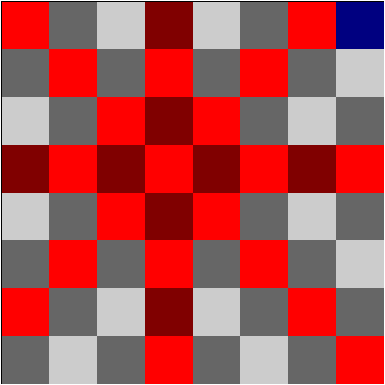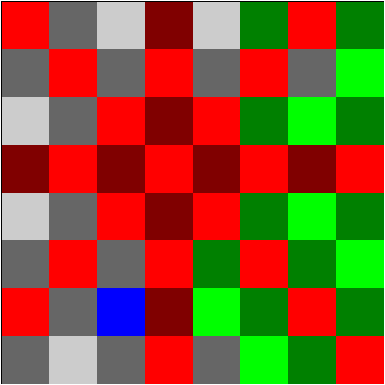# Funny Knight

I’ve stumbled upon a websited called Ain’t it funny how the knight moves?, which poses a simple problem: Visit all squares of the chessboard, except those attacked (or occupied) by a queen on d5, using a knight starting from h8.You cannot land on any of the red squares; you can visit any other square multiple times.

## First attempt

The language most suitable for this is obviously Prolog. First let’s define valid positions: both coordinates are between 1 and 8, and excluding the red squares:

``````valid(X-Y) :-
between(1, 8, X), between(1, 8, Y),
X =\= 4, Y =\= 5,
X + Y =\= 9, Y - X =\= 1.``````

Now we can define a valid move as

``````knight(1, 2). knight(1, -2). knight(-1, 2). knight(-1, -2).
knight(2, 1). knight(2, -1). knight(-2, 1). knight(-2, -1).

move(X-Y, X1-Y1) :-
knight(DX, DY),
X1 is X + DX, Y1 is Y + DY,
valid(X1-Y1).``````

The core of the solver will be the `jump/4` rule. It keeps track of the current position, the already visited squares, and the current path:

``````jump(_, Visited, Path, Path) :- length(Visited, 36), !.
jump(Pos, Visited, Path, Solution) :-
move(Pos, Pos1),
jump(Pos1, Visited1, [Pos1|Path], Solution).``````

The first rule says that when we have already visited all 36 goal squares, the solution (last parameter) will be the current path. The second rule says that we try to move to a new position (`Pos1`), add it to the list of `Visited` places if not yet visited, and continue jumping, adding the new position to the current path. Note that the path is built in reverse order.

The `add_new/4` rule also ensures that we do not visit a square again when nothing new was seen since the last visit:

``````add_new(Pos, Visited, Path, Visited) :-
member(Pos, Visited), !,
Visited = [Last|_],
tail(Pos, Path, Tail),
\+ member(Last, Tail).

The check is implemented in the first rule. When the position has already been visited, we check the part of the (reversed) path that starts at our last visit - this is computed by `tail/3`. If this contains the last visited square, it means that we haven’t visited anything new, so we are not allowed to make this move.

The `tail/3` rule is quite trivial:

``````tail(X, [X|T], [X|T]) :- !.
tail(X, [Y|Ys], T) :- X \= Y, tail(X, Ys, T).``````

Finally, we just call `jump/4` with suitable arguments, and reverse the solution:

``solve(X) :- jump(8-8, [8-8], [8-8], Path), reverse(Path, X).``

The solution is found in an instant, but it is quite long:

``````?- solve(X), length(X, N).
X = [8-8, 7-6, 8-4, 7-6, ...],
N = 257 ``````

That’s 256 moves after the initial position! Looks a bit too many, but it’s hard to tell by just these numbers. Let’s create some graphical output!

## Graphical output

We’ll use PostScript for the output, which can be then converted to PDF. The squares will be colored according to the following rules:

• the base color of invalid squares is red
• the base color of visited squares is green
• the base color of the current square is blue
• the base color of not yet visited squares is light grey
• light squares use the base color
• dark squares use half of each of the red/green/blue parametersThe main rule will be `show/1` that takes a path and prints one page for each state of the board. Using 50x50 point squares, the whole board fits nicely in a 500x500 page, so we first write the header:

``````show(Path) :-
write('%%BoundingBox: 0 0 500 500'), nl,
show(Path, []).``````

The last row calls `show/2`, where the second parameter is the list of already visited squares.

In PostScript, we can write the outline of a square by x y width height `rectstroke`, and fill a square by x y width height `rectfill`. The color can be set by red green blue `setrgbcolor`, where the parameters take values between 0 and 1.

On each page, we first draw the outline rectangle, then fill each square; the `showpage` command marks the end of the page. To loop on all squares we can use the builtin `forall` rule:

``````show([], _).
show([Current|Path], Seen) :-
write('50 50 400 400 rectstroke'), nl,
forall((between(1, 8, X), between(1, 8, Y)),
(
color(Current, Seen, X-Y, c(R,G,B)),
write(R), write(' '), write(G), write(' '), write(B),
write(' setrgbcolor '),
X1 is X * 50, Y1 is Y * 50,
write(X1), write(' '), write(Y1),
write(' 50 50 rectfill'), nl
)),
write(showpage), nl,
show(Path, [Current|Seen]).``````

The `color/4` rule determines the color based on the square type:

``````color(Current, Seen, X-Y, Color) :-
base_color(Current, Seen, X-Y, c(R,G,B)),
( (X + Y) mod 2 =:= 0 ->
R1 is R / 2, G1 is G / 2, B1 is B / 2,
Color = c(R1,G1,B1)
; Color = c(R,G,B)
).

base_color(_, _, Pos, c(1,0,0)) :- \+ valid(Pos).
base_color(Current, _, Current, c(0,0,1)).
base_color(_, Seen, Pos, c(0,1,0)) :- member(Pos, Seen).
base_color(_, _, _, c(0.8,0.8,0.8)).``````

Generating the commands, I put them in a file called `knight.ps`, then called GhostScript to convert it to PDF:

``\$ gs -o knight.pdf -sDEVICE=pdfwrite -g5000x5000 knight.ps``

Here is the output. As I suspected, there is a lot of unnecessary jumping, because the solver always tries the moves in a predefined sequence (according to the `knight/2` rule).

## Second attempt

A better approach may be to use a greedy algorithm: always find the closest new (not visited) square! In order to do this, we first try to find a square 1 jump away, then 2 and so on, until one is found:

``````find_closest(Pos, Visited, Solution) :-
between(1, 36, N),
find_in(N, Pos, Visited, [], Solution).``````

The `find_in/5` rule tries to find a new square in `N` jumps (the first parameter).

``````find_in(_, Pos, Visited, Path, Path) :-
\+ member(Pos, Visited), !.
find_in(0, _, _, _, _) :- !, fail.
find_in(N, Pos, Visited, Path, Solution) :-
N > 0, N1 is N - 1,
move(Pos, Pos1),
find_in(N1, Pos1, Visited, [Pos1|Path], Solution).``````

Now we only need to update the `jump/4` rule to use `find_closest/3`:

``````jump(_, Visited, Path, Path) :- length(Visited, 36), !.
jump(Pos, Visited, Path, Solution) :-
find_closest(Pos, Visited, [Pos1|Path1]),
append([Pos1|Path1], Path, Path2),
jump(Pos1, [Pos1|Visited], Path2, Solution).``````

The second rule says that we find the path to the closest new square - the new square is `Pos1`; the path leading to it (but not including it) is `Path1`. We append the old path to the new one, and continue to jump recursively.

Now the solution consists of only 58 moves! That’s good enough for me, although it may not be optimal.

## The whole program

``````valid(X-Y) :-
between(1, 8, X), between(1, 8, Y),
X =\= 4, Y =\= 5,
X + Y =\= 9, Y - X =\= 1.

knight(1, 2). knight(1, -2). knight(-1, 2). knight(-1, -2).
knight(2, 1). knight(2, -1). knight(-2, 1). knight(-2, -1).

move(X-Y, X1-Y1) :-
knight(DX, DY),
X1 is X + DX, Y1 is Y + DY,
valid(X1-Y1).

jump(_, Visited, Path, Path) :- length(Visited, 36), !.
jump(Pos, Visited, Path, Solution) :-
find_closest(Pos, Visited, [Pos1|Path1]),
append([Pos1|Path1], Path, Path2),
jump(Pos1, [Pos1|Visited], Path2, Solution).

find_closest(Pos, Visited, Solution) :-
between(1, 36, N),
find_in(N, Pos, Visited, [], Solution).

find_in(_, Pos, Visited, Path, Path) :-
\+ member(Pos, Visited), !.
find_in(0, _, _, _, _) :- !, fail.
find_in(N, Pos, Visited, Path, Solution) :-
N > 0, N1 is N - 1,
move(Pos, Pos1),
find_in(N1, Pos1, Visited, [Pos1|Path], Solution).

solve(X) :- jump(8-8, [8-8], [8-8], Path), reverse(Path, X).

% PostScript output

show(Path) :-
write('%%BoundingBox: 0 0 500 500'), nl,
show(Path, []).

show([], _).
show([Current|Path], Seen) :-
write('50 50 400 400 rectstroke'), nl,
forall((between(1, 8, X), between(1, 8, Y)),
(
color(Current, Seen, X-Y, c(R,G,B)),
write(R), write(' '), write(G), write(' '), write(B),
write(' setrgbcolor '),
X1 is X * 50, Y1 is Y * 50,
write(X1), write(' '), write(Y1),
write(' 50 50 rectfill'), nl
)),
write(showpage), nl,
show(Path, [Current|Seen]).

color(Current, Seen, X-Y, Color) :-
base_color(Current, Seen, X-Y, c(R,G,B)),
( (X + Y) mod 2 =:= 0 ->
R1 is R / 2, G1 is G / 2, B1 is B / 2,
Color = c(R1,G1,B1)
; Color = c(R,G,B)
).

base_color(_, _, Pos, c(1,0,0)) :- \+ valid(Pos).
base_color(Current, _, Current, c(0,0,1)).
base_color(_, Seen, Pos, c(0,1,0)) :- member(Pos, Seen).
base_color(_, _, _, c(0.8,0.8,0.8)).``````